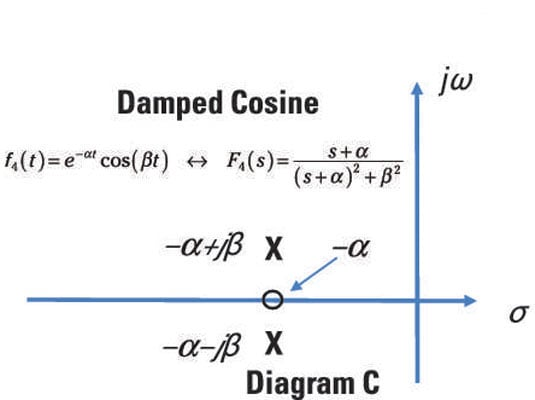##### Circuit Analysis For Dummies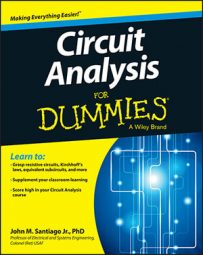Laplace transforms can be used to predict a circuit's behavior. The Laplace transform takes a time-domain function f(t), and transforms it into the function F(s) in the s-domain. You can view the Laplace transforms F(s) as ratios of polynomials in the s-domain. If you find the real and complex roots (poles) of these polynomials, you can get a general idea of what the waveform f(t) will look like.

For example, as shown in this table, if the roots are real, then the waveform is exponential. If they’re imaginary, then it’s a combination of sines and cosines. And if they’re complex, then it’s a damping sinusoid.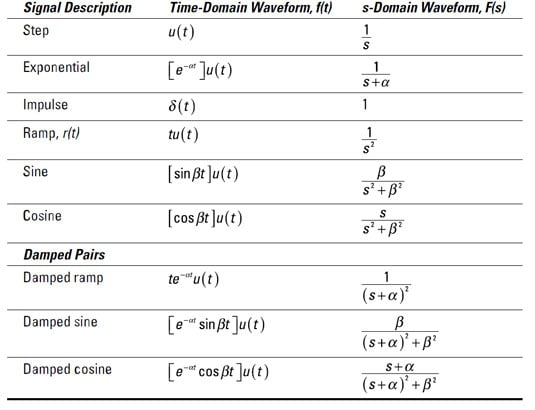The roots of the polynomial in the numerator of F(s) are zeros, and the roots of the polynomial in the denominator are poles. The poles result in F(s) blowing up to infinity or being undefined — they’re the vertical asymptotes and holes in your graph.

Usually, you create a pole-zero diagram by plotting the roots in the s-plane (real and imaginary axes). The pole-zero diagram provides a geometric view and general interpretation of the circuit behavior.

For example, consider the following Laplace transform F(s):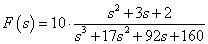This expression is a ratio of two polynomials in s. Factoring the numerator and denominator gives you the following Laplace description F(s):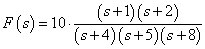The zeros, or roots of the numerator, are s = –1, –2. The poles, or roots of the denominator, are s = –4, –5, –8.

Both poles and zeros are collectively called critical frequencies because crazy output behavior occurs when F(s) goes to zero or blows up. By combining the poles and zeros, you have the following set of critical frequencies: {–1, –2, –4, –5, –8}.

This pole-zero diagram plots these critical frequencies in the s-plane, providing a geometric view of circuit behavior. In this pole-zero diagram, X denotes poles and O denotes the zeros.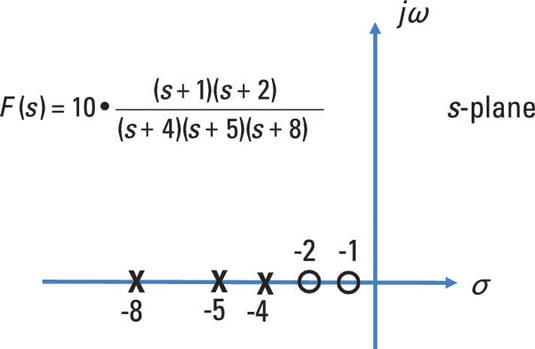Here are some examples of the poles and zeros of the Laplace transforms, F(s). For example, the Laplace transform F1(s) for a damping exponential has a transform pair as follows: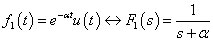The exponential transform F1(s) has one pole at s = –α and no zeros. Here, you see the pole of F1(s) plotted on the negative real axis in the left half plane.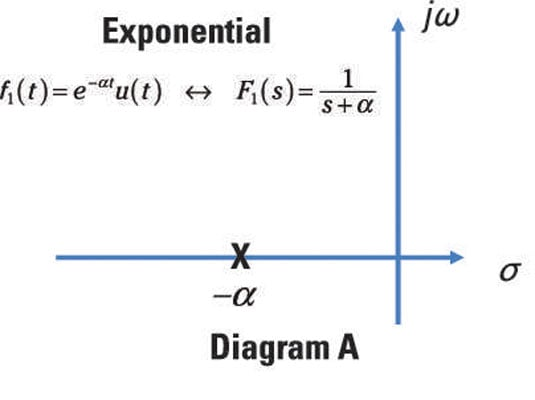The sine function has the following Laplace transform pair: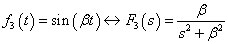The preceding equation has no zeros and two imaginary poles — at s = +jβ and s = –jβ. Imaginary poles always come in pairs. These two poles are undamped, because whenever poles lie on the imaginary axis jω, the function f(t) will oscillate forever, with nothing to damp it out. Here, you see a plot of the pole-zero diagram for a sine function.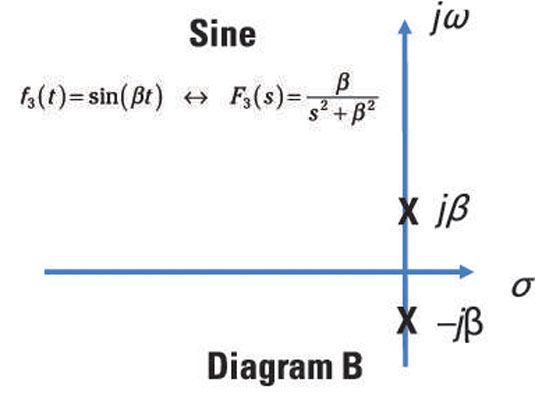A ramp function has the following Laplace transform pair: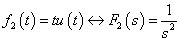The ramp function has double poles at the origin (s = 0) and has no zeros.

Here’s a transform pair for a damped cosine signal: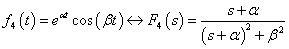The preceding equation has two complex poles at s = α + jβ and s = α – jβ and one zero at s = –α.

Complex poles, like imaginary poles, always come in pairs. Whenever you have a complex pair of poles, the function has oscillations that will be damped out to zero in time — they won’t go on forever. The damped sinusoidal behavior consists of a combination of an exponential (due to the real part α of the complex number) and sinusoidal oscillator (due to the imaginary part β of the complex number).

Here, you see depicted the pole-zero diagram for a damped cosine.